# Simple Particle Collisions Using a Grid

In this case an attempt is made to produce a box that will contain many particles, each a sphere of the same diameter and mass. The idea is for the particles to move around colliding with each other and the walls of the box, with the speed for each particle along its x axis, along its y axis and along its z axis being equal to the diameter of the particle or zero and for collisions to only take place if particles just touch the walls or each other.

It turns out that such a system is not possible when the particles are to obey the Newtonian physical laws for colliding spheres.

Other systems are produced, fitting the above conditions except for the rules for colliding.

## The Grid

Take a grid of cubes with the origin cube having its centre at the world origin. Each cube will have a side width of 2r, where r is the radius of each particle. The position of any particle will be the centre of the cube it is occupying. The conditions for the speed of the particles means they each will lie wholly within a cube.

## The Collisions

When a particle hits the wall then its velocity perpendicular to the wall will be reversed.

When two particles collide in a perfectly elastic collision the Newtonian laws of physics give that their velocities along the line joining their centres are exchanged and they retain their velocities tangential to this line.

## The Walls

For a particle to just touch a wall the wall position must be of the form (2p + 1)r, p an integer.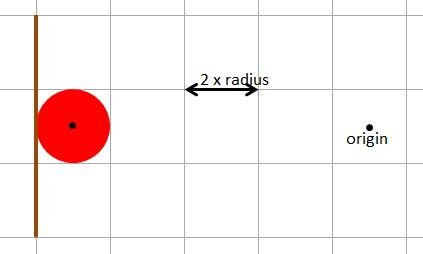Fig 9

## The Particles

Now having constrained the positions of the walls to ensure that the particles rebound on just touching them can the same be done for the particles themselves?

If the particles have an odd number of cubes between them they will meet only when overlapping completely. To ensure they meet in a touching position they need to have an even number of cubes between them, as in Fig 10.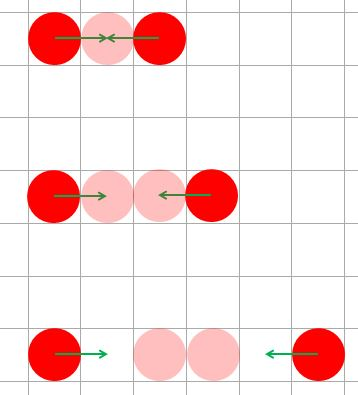Fig 10

Take two particles a distance 4 cubes apart in the x direction and 2 in the y direction, one travelling upwards and the other to the left, as in Fig 11.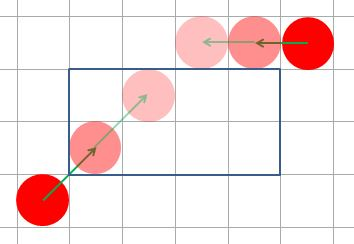Fig 11

After two frames it is clear that the two particles would collide. The first issue is that they do not actually touch or intersect. There are two ways to overcome this. One way is to make the diameter of the particle equal to the length of a diagonal across the cube. The second is to state that a collision takes place when the distance between the positions of two particles is less than or equal to the length of a diagonal of the cube.

The second issue is more subtle and relates to the velocities of the particles after the collision. Fig 12 shows the velocities and positions before and after a collision.Fig 12

Consider a situation when two particles collide and obey the Newtonian rules.

The green arrows show the velocities before and after the collision and the blue arrows the components along and at right angles to the line of collision.

Note that after the collision the speeds no longer obey the initial rules of one cube distance along the x, y or z axes per frame and keep inside the cubes. So any attempt to maintain collisions only when the particles touch is fruitless. Also note that the vertical speed of the top particle has increased. The maximum possible vertical speed (or horizontal speeds) is 2√3 r, the diagonal speed of a particle. At this speed and with no control over positioning a particle can escape the box.

Systems where the Newtonian laws of physics are obeyed is considered [TBA]. This page now considers other physics.

## Other Physics

Two simple physics could be:

1. Colliding particles swap velocities.
2. Colliding particles reverse velocities.

These at least ensure that speeds in the x, y and z directions are maintained and so particles cannot escape the box.

What about collisions between paticles in these cases? To ensure contact, by just touching alone, then we have already seen that there must be an even number of cubes between them. Placing particles in the box so this conditions exists is not straightforward so simplify this to - every particle has two cubes between them. This means that placing a particle at the cube origin and counting cube positions from this point every cube with position of the form (3m, 3n, 3p), and only these cube positions, has a particle in it. Should one particle be missing then there will be two particles with an odd number of cubes between them which could meet by overlapping, as in Fig 13.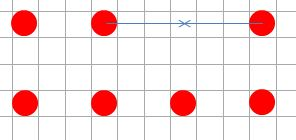Fig 13

However setting random velocities to such particles could lead to a situation such as in Fig 14 which would again lead to particles totally overlapping.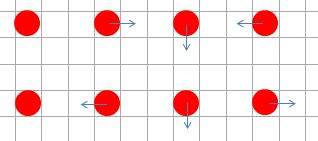Fig 14

Keeping the speed restrictions in the x, y and z directions to 2r and dropping the condition that particles can only make contact by touch and letting them fully overlap allows us to place the particles in any random cube. Movement is still within the grid cubes and particles will touch but not overlap the walls of the box.

## Collision Conditions

One condition already stated above is that the distance between the positions of two particles is less than or equal to the length of a diagonal of the cube which is 2√3 r.

A second condition is that the particles must be travelling towards each other. By considering relative velocities one particle can be considered as still. The component of velocity for the moving particle along the line joining their centres will be positive or negative depending weather it is moving away from or towards the other. The magnitude of the component velocity is found by using the dot product of their relative positions and relative velocities.

## Creating The Examples

The walls of the box are positioned using (2p + 1)r, for an integer p. Two parallel walls will be positioned at (-2p + 1)r and (2p + 1), p > 0.

Particles can be positioned 2jr, where j is an integer with -p <= j <= p

To generate j randomly, use floor(1 - 2 * random)

In the Playground code spaceMultiplier is used as the p.

The speed of all particles in the x, y and z directions is 2r or 0. For the particle velocity in each direction the speed will therefore be multiplied by -1, 0 or 1. The numbers -1, 0, and 1 can be generated by using floor(2.99 * random - 1).

## Playground Examples

In both these examples on particle is coloured red to aid focus on one particle if needed. Also by removing the comment markers, lines 140 and 144, in the swap example and lines137 and 141, in the reverse example you can see the path trace of the red particle.

Which one do you think gives the most random type movement?

PG: Particles Swap Velocities
PG: Particles Reverse velocity